American in Spain

# Distance to the Horizon

March 25, 2008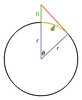A couple years ago, a friend and I, while staying at a beachside condo, were standing on the balcony staring at the flat, distant horizon, and we got to wondering, "I wonder how far we can see right now?" and "I wonder how much further we can see up here on the 9th floor than the people out there on the beach?" Unable to resist, I got out a pen and paper and started drawing circles and right triangles, and we quickly had our answer. I was pleased that I still had the trigonometry knowledge to figure out the answer to such a question.

So hold on tight, because here we go, on a trigonometric journey to the end of the Earth! First of all, we have to define what we mean by "How far to the horizon?" The simplest way, and the most common answer to the question if you ask Google, is to calculate the distance from the observer's eyes to the horizon. This is a simple pythagorean triangle calculation. But what I mean, and what makes the most sense to me is to rephrase the question to, "How far would I have to travel along the surface of the earth to get to the last point I can see on the horizon?" That question is slightly trickier to solve. So let's get going...

### Diagram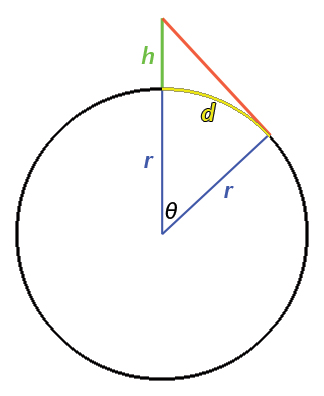Obviously this diagram is not drawn to scale. Let's examine the components of the diagram:

r

h

height/altitude of observer

d

distance along the Earth to visible horizon

So, given r and h, we want to calculate d.

### Calculation

We know from trigonometry that the cosine of an angle of a right triangle is equal to the length of the adjacent side over the length of the hypotenuse.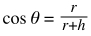So if we isolate theta...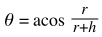Trigonometry also tells us that the length of an arc is the angle, in radians, of the arc multiplied by the radius.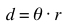So the final equation for the length of d in terms of h and r is: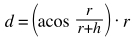If we plot this equation, plugging in the radius of the Earth, and dividing by 1000 to scale the y-axis to kilometers while the x-axis remains in meters, we get: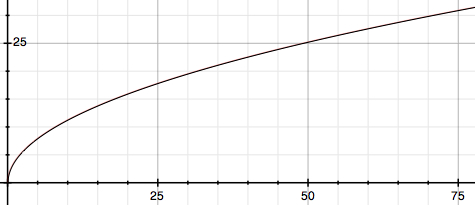If we go up to an altitude of 50 meters, we can see 25 kilometers out to the horizon. This does, in fact, turn out to be extremely close to the square root of r / r + h that the Pythagorean theorem gives us for the length of the red line in the diagram. Only at very large values of h do the plotted lines (the length of the red line vs. the length of the yellow arc) diverge much.

### Data Points

Let's look at some concrete data points:

Meters Altitude

Kilometers To Horizon

1

3.572

2

5.051

3

6.186

4

7.143

5

7.986

6

8.749

7

9.450

8

10.102

9

10.715

10

11.294

In a chart, these numbers look like: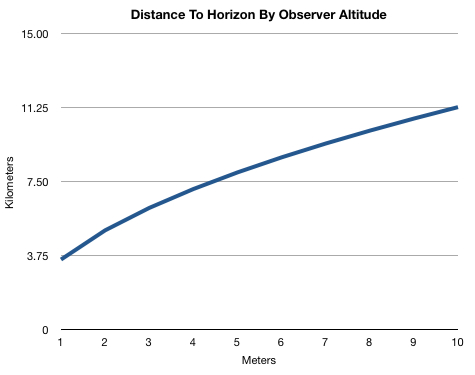This means that a 200-cm-tall adult can see 1.5 km further than a 100-cm-tall child.

### English System

For the metrically ignorant Americans, we'll give the same data in terms they can understand:

Feet Altitude

Miles To Horizon

3

2.12

6

3.00

9

3.68

12

4.24

15

4.75

18

5.20

21

5.61

24

6.00

27

6.37

30

6.71

And in a chart: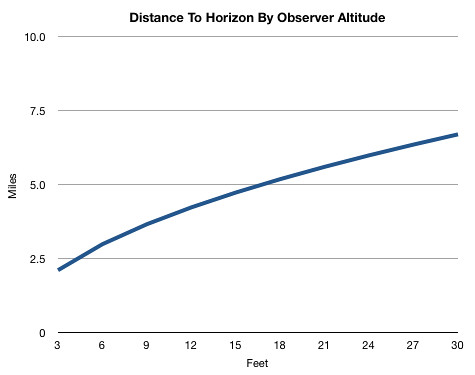So a 6-foot-tall adult, standing on the beach, can see exactly 3 miles to the horizon. You can see why, when looking for land, it's advantageous to have someone looking out from a crow's nest. At only 24 feet up, the visible distance doubles to 6 miles!

### Distance To Horizon Calculator

Units

MetricEnglish

Height or Altitude

meters

Distance to Horizon

0

kilometers